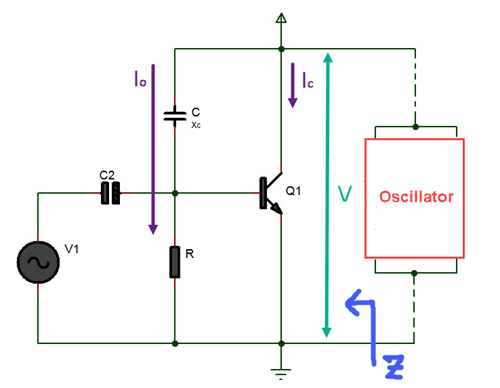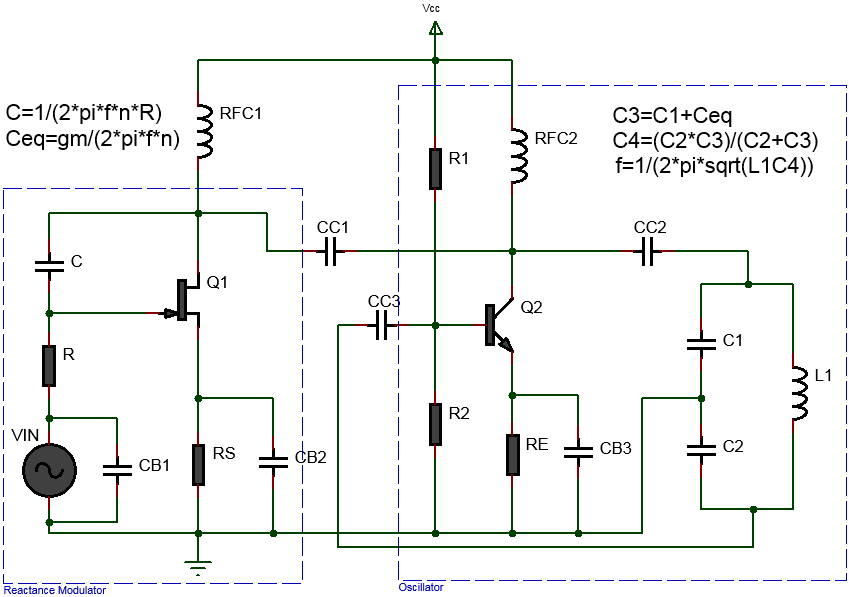# Reactance Modulator Calculator

BJT Reactance Modulator Calculator
Inputs:BJT Reactance Modulator Calculator

A reactance modulator works by changing the impedance of an oscillator circuit. When a voltage is applied to the circuit, it changes the reactance of the circuit, which in turn changes the frequency of the oscillator. Thus, a reactance modulator can be used to change the frequency of an oscillator. The above online BJT Reactance Modulator Calculator helps you to calculate the capacitor value, resistor value and equivalent capacitance of a BJT transistor based reactance modulator.

Equations Used:
$$C_{eq} = g_mCR$$
$$C=\frac{1}{n 2 \pi f R}$$
$$X_c = nR$$(Xc>>R)
FET Reactance Modulator Calculator Calculator
Inputs:$$C_{eq} = g_mCR$$
$$C=\frac{1}{n 2 \pi f R}$$
$$X_c = nR$$(Xc>>R)